# Maharashtra Board Class 11 Chemistry Important Questions Chapter 8 Elements of Group 1 and 2

Balbharti Maharashtra State Board 11th Chemistry Important Questions Chapter 8 Elements of Group 1 and 2 Important Questions and Answers.

## Maharashtra State Board 11th Chemistry Important Questions Chapter 8 Elements of Group 1 and 2

Question 1.
Why is hydrogen studied separately even though it appears at the top of group 1?
Even though hydrogen appears at the top of group 1 containing alkali metals, it is studied separately because many of its properties differ from that of the alkali metals.

Question 2.
Give reason: Hydrogen (H2) molecule is also referred to as dihydrogen.

• The nucleus of a hydrogen atom consists of one positively charged proton i.e., a nuclear charge of +1, and one extranuclear electron.
• As this electron is indirect influence of nuclear attraction, hydrogen has a little tendency to lose this electron.
• However, it can easily pair with the other electron forming a covalent bond.
• Therefore, it exists in diatomic form as H2 molecule and hence, it is also referred to as dihydrogen.

Question 3.
Why does hydrogen occur in a diatomic form rather than in monoatomic form under normal
conditions?

• Hydrogen atom has only one electron in its valence shell having electronic configuration 1s1.
• It can acquire stable configuration of helium by sharing this electron with another hydrogen atom.
• Therefore, it shares its single electron with electron of the other H-atom to achieve stable inert gas configuration of He.
• Thus, hydrogen readily forms diatomic molecule and exists as H2 rather than in monoatomic form.Question 4.
Write a note on occurrence of hydrogen.

• In the free state hydrogen exists as dihydrogen gas.
• Hydrogen is most abundant element in the universe and constitutes 70% of the total mass of the universe.
• Hydrogen is also the principal element in the solar system.
• On the earth, hydrogen is the tenth most abundant element on mass basis and the third most abundant element on atom basis.

Question 5.
State whether the following statements are TRUE or FALSE. Correct the false statement.
1. Electronic configuration of hydrogen is 1s1.
ii. H+ ion formed by loss of the electron from hydrogen atom exists freely.
iii. H+ is nothing but a proton.
iv. Metastable metallic hydrogen was discovered at Harvard university, USA, in January 2017.
i. True
ii. False
Hydrogen atom does not exist freely and is always associated with other molecules i.e., H3O+.
iii. True
iv. True

Question 6.
Explain the laboratory methods for preparation of dihydrogen.
Laboratory methods for preparation of dihydrogen:
i. By action of dilute HCl on zinc granules: Zinc granules on reaction with dilute hydrochloric acid (HCl) liberates hydrogen gas.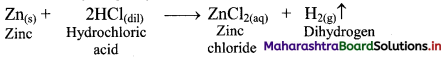ii. By action of aqueous NaOH on zinc: Zinc on reaction with aqueous sodium hydroxide (NaOH) forms soluble sodium zincate and liberates hydrogen gas.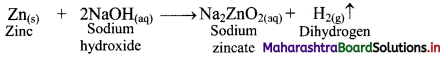Question 7.
Describe the industrial method of preparation of dihydrogen by electrolysis of pure water.
i. Pure water is a poor conductor of electricity. Therefore, a dilute aqueous solution of acid or alkali is used to prepare dihydrogen by electrolysis.
ii. For example, electrolysis of dilute aqueous solution of sulphuric acid yields two volumes of hydrogen at cathode and one volume of oxygen at anode.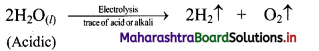Question 8.
How is pure dihydrogen (> 99.5% purity) gas obtained from barium hydroxide?
Electrolysis of warm aqueous solution of barium hydroxide using nickel electrodes yields pure dihydrogen (> 99.5% purity).Question 9.
Explain the terms:
i. Syngas
ii. Water-gas shift reaction.
i. Syngas:

• Syngas is the mixture of CO and H2. It is also called ‘water-gas’.
• It is used for the synthesis of CH3OH and many hydrocarbons, hence, the name syngas or ‘synthesis gas’.
• Production of syngas is also the first stage of gasification of coal.

ii. Water-gas shift reaction:
The carbon monoxide in the water-gas is transformed into carbon dioxide by reacting with steam in presence of iron chromate as catalyst.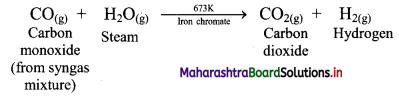This reaction is called water-gas shift reaction.

Question 10.
Complete the following chemical reactions: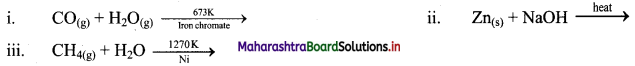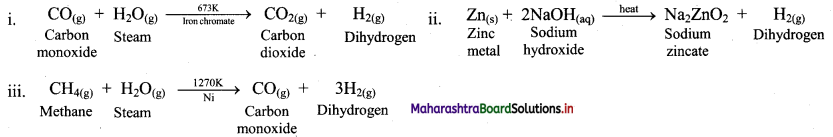Question 11.
Enlist physical properties of dihydrogen.
Physical properties of dihydrogen:

• Dihydrogen is a colourless, tasteless and odourless gas.
• It bums with a pale blue flame.
• It is a nonpolar and water-insoluble gas.
• It is lighter than air.

Question 12.
What is the action of dihydrogen on the following?
i. Metals
ii. Dioxygen
i. Action of dihydrogen on metals:
a. Dihydrogen combines with all the reactive metals including alkali metals, calcium, strontium and barium at high temperature to form metal hydrides.
b. For example: Dihydrogen combines with metallic sodium at high temperature to yield sodium hydride.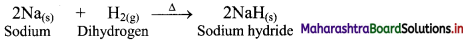ii. Action of dihydrogen on dioxygen: Dihydrogen reacts with dioxygen in the presence of catalyst or by heating to form water. This reaction is highly exothermic.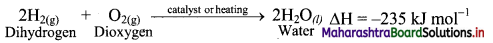Question 13.
Explain the effect of high bond dissociation energy of H-H bond on chemical reactivity of dihydrogen?

• The bond dissociation energy of H-H bond is very high i.e, 436 kJ mol-1. and thus, it does not react easily under normal conditions.
• However, at high temperature or in the presence of catalysts, hydrogen combines with many metals and non-metals to form corresponding hydrides and halides respectively.Question 14.
What happens when dihydrogen reacts with halogens?
i. Dihydrogen reacts with halogens (X2) to give the corresponding hydrogen halides (HX).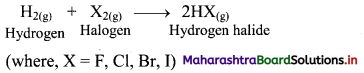ii. Dihydrogen reacts with fluorine to form hydrogen fluoride even at very low temperature (-250°C) in dark.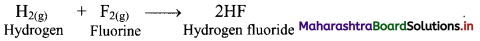iii. However, the reaction with iodine requires a catalyst as the vigour of reaction of dihydrogen decreases with increasing atomic number of halogen.

Question 15.
Explain the reducing nature of hydrogen with chemical reactions.
Dihydrogen reduces oxides and ions of some metals that are less reactive than iron, to the corresponding number of halogen metals at moderate temperature.
e.g.
i. CuO(s) + H2(g) → Cu(s) + H2O(l)
ii. Fe3O4(s) + 4H2(g) → 3Fe(s) + 4H2O(s)
iii. Pd2+(aq) + H2(g) → Pd(s) + 2H+(aq)

Question 16.
What is hydrogenation?
Hydrogenation is the reaction in which hydrogen gas reacts with unsaturated organic compounds in the presence of a catalyst to form hydrogenated (saturated) compounds.

Question 17.
How does dihydrogen react with various organic compounds to give useful, commercially important products?
i. Hydrogenation of unsaturated organic compounds:
e.g. Hydrogenation of unsaturated organic compounds such as vegetable oil using nickel catalyst gives saturated organic compounds such as solid edible fats like vanaspati ghee.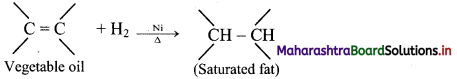ii. Hydroformylation of olefins and subsequent reduction of aldehyde to form alcohol: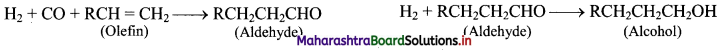Question 18.
Explain hydroformylation reaction of olefins using a suitable example.
Hydroformylation of olefins gives aldehydes which on further reduction gives alcohols.
e.g. i. Hydroformylation of propene gives butyraldehyde.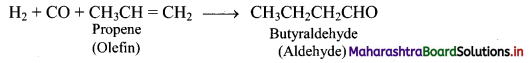ii. Butyraldehyde further undergoes reduction to give n-butyl alcohol.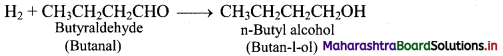Question 19.
What are the uses of dihydrogen?
Dihydrogen is used in

• the production of ammonia.
• the formation of vanaspati ghee by catalytic hydrogenation of oils.
• rocket fuel (mixture of liquid hydrogen and liquid oxygen).
• the preparation of important organic compounds like methanol in bulk quantity.
$$2 \mathrm{H}_{2(\mathrm{~g})}+\mathrm{CO}_{(\mathrm{g})} \stackrel{\text { Cobaltcatalyst }}{\longrightarrow} \mathrm{CH}_{3} \mathrm{OH}_{(l)}$$
• the preparation of hydrogen chloride (HCl) and metal hydrides.Question 20.
Justify the placement of hydrogen in the group of alkali metals with the help of reaction with halogens.
i. Hydrogen on reaction with halogens (X2) give compounds with general formula HX.
e.g. H2 + Cl2 → 2HCl
ii. Similarly, alkali metals (M) on reaction with halogens (X2) give compounds with general formula MX.
e.g. 2Na + Cl2 → 2NaCl
iii. Thus, H2 and alkali metals are monovalent elements and more electropositive than halogens. This similarity justifies the position of hydrogen in the group 1.

Question 21.
What do you mean by s-block elements? Where are they placed in the modern periodic table?

• Elements of group 1 and group 2 in which the last electron enters into ‘ns’ subshell are s-block elements.
• The s-block elements are placed on the extreme left in the modem periodic table.

Question 22.
Name elements of group 1 and group 2.

• Group 1 of the periodic table consists of the elements: hydrogen, lithium, sodium, potassium, rubidium, caesium and francium.
• Group 2 of the periodic table consists of elements: beryllium, magnesium, calcium, strontium, barium and radium.

Question 23.
What are alkali metals?
The elements of group 1 except hydrogen are collectively called alkali metals.

Question 24.
What are alkaline earth metals?
The elements of group 2 are collectively called alkaline earth metals because they occur as minerals in rocks.Question 25.
Write a note on occurrence of group 1 and group 2 elements:
i. Group 1 (alkali metals):

• Two elements of group 1 i.e., sodium and potassium are the sixth and seventh most abundant elements present in the earth’s crust.
• However, francium does not occur appreciably in nature because it is radioactive and has short half-life period.

ii. Group 2 (alkaline earth metals):

• The elements magnesium and calcium are found abundantly in earth’s crust.

Question 26.
Give reasons: s-block elements are never found in free state in nature.

• s-Block elements contain group 1 and group 2 elements.
• The general outer electronic configuration of the group 1 elements is ns1 and that of the group 2 elements is ns2.
• The loosely held s-electrons in the valence shell of these elements can be easily removed to form metal ions.
• As a result, they are highly reactive in nature and always found in combined state.

Hence, s-block elements are never found in free state in nature.

Note: Electronic configurations of group 1 elements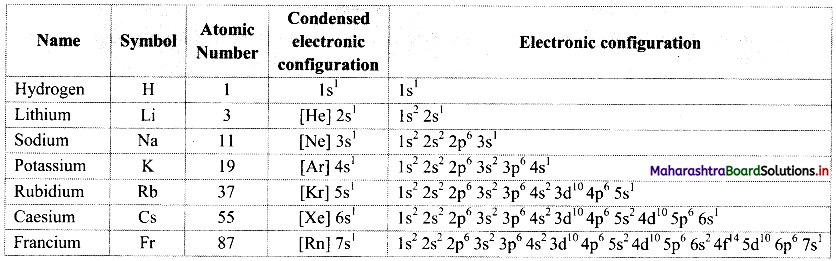Note: Electronic configurations of group 2 elements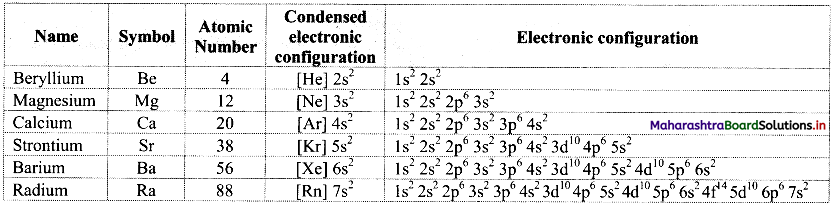Question 27.
Describe the physical properties of alkali and alkaline earth metals.

• All the alkali and alkaline earth metals are silvery white in appearance.
• Due to their large atomic size they have low density.
• Both alkali and alkaline earth metals are soft, however, alkaline earth metals are harder than the alkali metals.
• Alkali metals are the most electropositive elements while alkaline earth metals are comparatively less electropositive than alkali metals.

Question 28.
Explain why do the group 1 and group 2 elements form diamagnetic and colourless compounds.

• Unipositive ions of all the elements of group 1 have inert gas configuration and hence, they have no unpaired electron.
• Similarly, group 2 elements can lose their two valence shell electrons and form divalent ions that have inert gas configuration with no unpaired electrons.

Hence, due to the absence of unpaired electrons, compounds formed by group 1 and group 2 elements are diamagnetic and colourless.Question 29.
Why do the properties of lithium and beryllium differ from the rest of the group 1 and group 2 elements?
The properties of lithium and beryllium differ from the rest of the group 1 and group 2 elements due to their extremely small size and comparatively high electronegativity.

Question 30.
Complete the following table.

 Group 1 elements Group 2 elements ………………. Alkaline earth metals Outer electronic configuration: ………………. Outer electronic configuration: ns2 Monovalent positive ions ……………….

 Group 1 elements Group 2 elements Alkali metals Alkaline earth metals Outer electronic configuration: ns1 Outer electronic configuration: ns2 Monovalent positive ions Divalent positive ions

Question 31.
State the trends in the following properties of group 1 and group 2 elements down a group.
iii. Ionization enthalpy
iv. Electronegativity
v. Standard reduction potential

 Sr. no. Property Down a group i. Atomic radii Increases ii. Ionic radii Increases iii. Ionization enthalpy Decreases iv. Electronegativity Decreases V. Standard reduction potential Decreases

Question 32.
Give reasons: Potassium superoxide is used in breathing equipment used for mountaineers and in submarines and space.
i. Potassium superoxide has ability to absorb carbon dioxide and give out oxygen at the same time.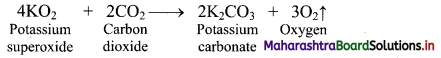ii. Due to this property of KO2, it is used in breathing equipment used for mountaineers and in submarines and space.Question 33.
What is the oxidation state of:
i. Na in Na2O2?
ii. K in KO2?
i. Oxidation state of Na in sodium peroxide (Na2O2):
Let x be the oxidation state of Na in Na2O2.
The net charge on peroxide ion $$\left(\mathrm{O}_{2}^{2-}\right)$$ is -2.
Since any compound is electrically neutral, it has an overall charge as zero.
∴ 2x + (-2) = 0
∴ x = + 1
∴ Oxidation state of Na in Na2O2 is +1.

ii. Oxidation state of K in potassium dioxide/potassium superoxide (KO2):
Let x be the oxidation state of K in KO2
The net charge on superoxide ion $$\left(\mathrm{O}_{2}^{-}\right)$$ is -1.
Since any compound is electrically neutral, it has an overall charge as zero.
∴ x + (-1) = 0
x = + 1
∴ Oxidation state of K in KO2 is + 1.
[Note: Oxidation state of alkali metal is always +1.]

Question 34.
Magnesium strip slowly tarnishes on keeping in air but metallic calcium is readily attacked by air. Explain.

• The reactivity of group 2 metals increases with increasing atomic radius and lowering of ionization enthalpy
down the groups.
• Thus, calcium has lower ionization enthalpy. Therefore, calcium is more reactive than magnesium.
• Hence, Mg reacts slowly with air forming a thin film of oxide resulting into tarnishing, whereas Ca reacts readily at room temperature with oxygen and nitrogen in the air.

Question 35.
What happens when alkali metals react with hydrogen and halogens?
i. Reaction with hydrogen: Alkali metals react with hydrogen at high temperature to form the Corresponding metal hydrides.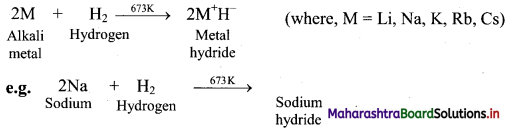ii. Reaction with halogens: All the alkali metals react vigorously with halogens to produce their ionic halide salts.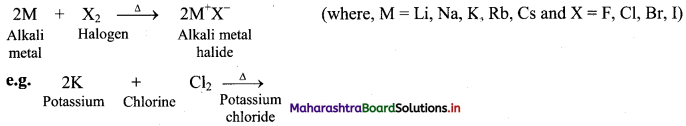[Note: As we move down the group, the reactivity of alkali metals towards hydrogen and halogens decreases in the following order: Li > Na > K > Rb > Cs.]

Question 36.
NaCl is an ionic compound but LiCl has some covalent character, explain.

• Li+ ion has very small size and therefore, the charge density on Li+ is high.
• Due to this, it has high tendency to distort the electron cloud around the negatively charged chloride ion (Cl) which is larger in size.
• This results in partial covalent character of the LiCl bond.
• Na+ ion cannot distort the electron cloud of Cl due to the bigger size of Na+ compared to Li+.

Hence, NaCl is an ionic compound but LiCl has some covalent character.Question 37.
Why is lithium iodide most covalent in nature among alkali halides?

• Among the alkali metal ions, Li+ ion is the smallest cation while among halides, anion I has the largest size.
• Thus, electron cloud around I ion is easily distorted by Li+ ion leading to polarisation of anion and covalency.
• Also, the difference in electronegativities of Li and I is small.

Hence, lithium iodide is most covalent in nature among alkali halides.

Question 38.
Explain the reactivity of alkaline earth metals towards:
i. Water
ii. Hydrogen
iii. Halogens
i. Reaction with water:
a. The elements of group 2 (alkaline earth metals) react with water to form metal hydroxide and evolve hydrogen gas.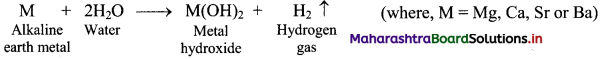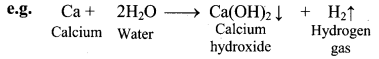b. Be does not react with water at all, Mg reacts with boiling water while Ca, Sr, Ba react vigorously even with cold water.

ii. Reaction with hydrogen: All alkaline earth metals except beryllium (Be), when heated with hydrogen form MH2 type hydrides.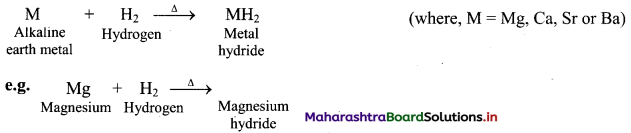iii. Reaction with halogens: All the alkaline earth metals combine with halogens at high temperature to form their corresponding halides.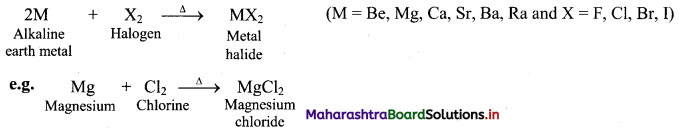[Note: As we move down the group, the chemical reactivity of alkaline earth metals increases in the order Mg < Ca < Sr < Ba.]

Question 39.
Complete the following chemical equations.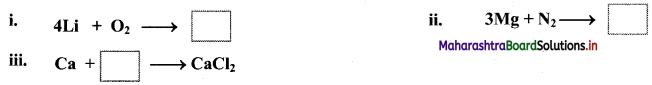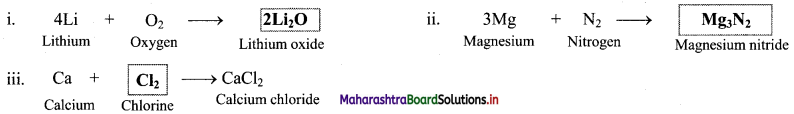Question 40.
Describe the reducing nature of group 1 and group 2 elements.
The reducing power of an element is measured in terms of standard electrode potential (E0) corresponding to the following transformation i.e, tendency to lose electron.
$$\mathrm{M}_{(\mathrm{s})} \longrightarrow \mathrm{M}_{(\mathrm{aq})}^{+}+\mathrm{e}^{-}$$
i. Reducing nature of group 1 elements:

• All the alkali metals have high negative values of E0 which indicates that they have strong reducing nature and hence, they can be used as strong reducing agents.
• Lithium is the most powerful and sodium is the least powerful reducing agent in the group.

ii. Reducing nature of group 2 elements:

• All the alkaline earth metals have high negative values of stanard reduction potential (E0) and are strong reducing agents.
• However, reducing power of alkaline earth metals is less than that of alkali metals.Question 41.
Explain the nature of the solution formed by group 1 and group 2 metals in liquid ammonia.
i. The alkali metals are soluble in liquid ammonia and thus, they dissolve in it giving deep blue solutions which are conducting in nature.
M + (x + y)NH3 → [M(NH3)x]+ + [e(NH3)y]
ii. The blue colour of the solution is due to the ammoniated electron.
iii. These solutions are paramagnetic and on standing slowly liberate hydrogen resulting in the formation of amide.
$$\mathrm{M}_{(\mathrm{am})}^{+}+\mathrm{e}^{-}+\mathrm{NH}_{3(l)} \longrightarrow \mathrm{MNH}_{2(\mathrm{am})}+\frac{1}{2} \mathrm{H}_{2(\mathrm{~g})}$$
(where ‘am’ denotes solution in ammonia.)
iv. As a result, the blue colour of the solution changes to bronze and the solution becomes diamagnetic.
v. Similarly, the alkaline earth metals are also soluble in liquid ammonia which give deep blue-black coloured solutions.
M + (x + 2y) NH3 → [M(NH3)x]2+ + 2[e(NH3)y]

Question 42.
Explain: Diagonal relationship in group 1 and group 2.

• Elements belonging to the same group are expected to exhibit similarity and gradation in their properties.
• However, first alkali metal, lithium, and the first alkaline earth metal, beryllium, do not fulfil this expectation.
• Thus, lithium shows many differences when compared with the remaining alkali metals and shows similarity with magnesium, the second alkaline earth metal.
• Similarly, beryllium shows many differences with remaining alkaline earth metals and shows similarity with aluminium, the second element of the next main group i.e., group 13.
• The relative placement of these elements with similar properties in the periodic table is across a diagonal and thus, it is called diagonal relationship.Question 43.
Explain diagonal relationship between lithium and magnesium with respect to:
i. Property of chlorides
ii. Thermal decomposition of their carbonates
Both lithium and magnesium show similarities in various physical and chemical properties as follows:
i. Property of chlorides: Chlorides of lithium (LiCl) and magnesium (MgCl2) are deliquescent as group 2 elements form deliquescent chlorides. These chlorides form corresponding hydrates (LiCl.2H2O and MgCl2.8H2O) on crystallization from their aqueous solutions.

ii. Thermal decomposition of carbonates: Heating of lithium carbonate and magnesium carbonate results in their easy decomposition to form corresponding oxides and carbon dioxide (CO2).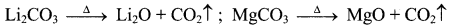Question 44.
Mention the properties of lithium that differ from rest of the alkali group metals.

• Reaction with nitrogen: Only lithium from alkali group metals reacts with nitrogen present in the air on heating, while rest of the members do not react with nitrogen.
• Thermal decomposition of carbonates: Alkali metal carbonates show no reaction on heating, while lithium carbonate decomposes on heating to form the corresponding oxide and liberate carbon dioxide gas.
• Property of chlorides: Lithium (LiCl) is deliquescent and forms corresponding hydrate (LiCl.2H2O). Other alkali chlorides are not deliquescent and do not form hydrates.Question 45.
Write a note on the diagonal relationship between Be and Al.
OR
What are the similarities between beryllium and aluminium?
i. Beryllium is placed in the group 2 and period 2 of the modem periodic table. It resembles aluminium which is placed in group 13 and period 3.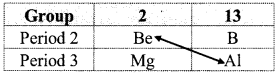ii. Due to nearly same charge to radius ratio of their ions, beryllium ($$\frac {2}{31}$$ = 0.065) and aluminium ($$\frac {3}{53.55}$$ = 0.056) exhibit diagonal relationship.
iii. Due to diagonal relationship, Be and Al show following similarities in their properties:
a. Nature of bonding: Both Be and Al have tendency to form covalent chlorides.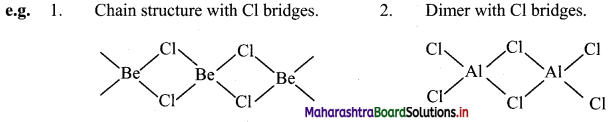b. Lewis acids: BeCl2 and AlCl3 act as Lewis acids.
c. Solubility in organic solvents: BeCl2 and AlCl3 are soluble in organic solvents.
d. Nature of oxide: Both Be and Al form amphoteric oxides.

Question 46.
Explain the amphoteric nature of aluminium oxide with the help of reactions.
Al2O3 (magnesium oxide) reacts with both acid (HCl) as well as base (NaOH) to form the corresponding products and therefore, it is amphoteric in nature.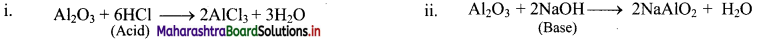Question 47.
Beryllium shows many differences with other alkaline earth metals. Discuss these differences with respect to chlorides and oxides.
1. Properties of chlorides: Beryllium chloride is covalent whereas chlorides formed by other alkaline earth metals are ionic in nature. Beryllium chloride is a strong Lewis acid whereas chlorides formed by other alkaline earth metals are not Lewis acids. Beryllium chloride is soluble in organic solvents whereas chlorides formed by other alkaline earth metals are insoluble in organic solvents.

2. Properties of oxide: Beryllium oxide is amphoteric whereas oxides formed by other alkaline earth metals are basic in nature.

Question 48.
Complete the following chemical equations.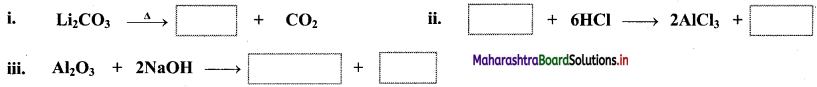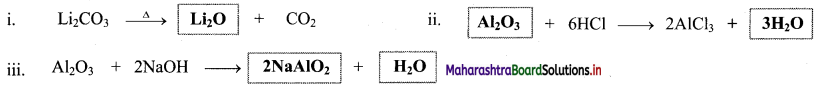Question 49.
Write the uses of
i. alkali metals
ii. alkaline earth metals
i. Uses of alkali metals:

• Lithium metal is used in long-life batteries used in digital watches, calculators and computers.
• Liquid sodium is used for heat transfer in nuclear power stations.
• Potassium chloride is used as a fertilizer.
• Potassium is used in manufacturing potassium superoxide (KO2) for oxygen generation. It is good absorbent of carbon dioxide.
• Caesium is used in photoelectric cells.

ii. Uses of alkaline earth metals:

• Beryllium is used as a moderator in nuclear reactors.
• Alloy of magnesium and aluminium is widely used as structural material and in aircrafts.
• Calcium ions are important ingredient in biological system, essential for healthy growth of bones and teeth.
• Barium sulphate is used in medicine as barium meal for intestinal X-ray.

Question 50.
State the importance of sodium and potassium in biological system.

• Sodium ion is present as the largest supply in all extracellular fluids. These fluids provide medium for transporting nutrients to the cells.
• The concentration of sodium ion in extracellular fluid regulates the flow of water across the membrane.
• Sodium ions participate in the transmission of nerve signals.
• Potassium ions are the most abundant ions within the cells. They are required for maximum efficiency in the synthesis of proteins and also in oxidation of glucose.Question 51.
How are the following ions of group 2 elements biologically important?
i. Mg2+
ii. Ca2+
i. Magnesium ion (Mg2+)

• Mg2+ ions are important part of chlorophyll in green plants.
• They play an important role in the breakage of glucose and fat molecules, synthesis of proteins with enzymes and regulation of cholesterol level.

ii. Calcium ion (Ca2+)

• Ca2+ ions are important for bones and teeth in the form of apatite [Ca3(PO4)2].
• They play an important role in blood clotting.
• Ca2+ ions are required for contraction and stretching of muscles.
• They are also required to maintain the regular beating of heart.

Question 52.
Explain Solvay process for manufacture of sodium carbonate.
Sodium carbonate (Na2CO3) is commercially prepared by Solvay process. Preparation of sodium carbonate by Solvay process involves two stages.
i. In the first stage of Solvay process, carbon dioxide gas is bubbled through a concentrated solution of NaCl which is saturated with NH3. This results in the formation of ammonium bicarbonate. Crystals of sodium bicarbonate separate as a result of the following reactions.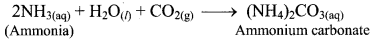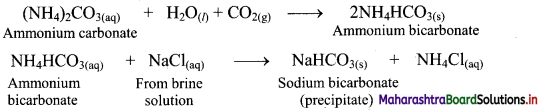ii. Ammonium bicarbonate and sodium chloride undergoes double decomposition reaction to form sodium bicarbonate. As sodium bicarbonate has low solubility, it precipitates out in the form of crystals.
iii. In the second stage, the separated crystals of sodium bicarbonate are heated to obtain sodium carbonate (Na2CO3).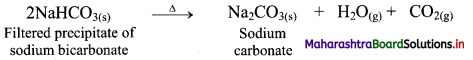iv. NH4Cl obtained in this process is treated with slaked lime, Ca(OH)2, to recover NH3 while CaCl2 is obtained as a byproduct.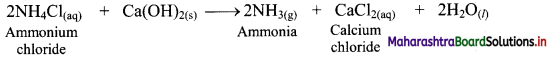Question 53.
How is ammonia recovered in Solvay process? Name the important by-product obtained in the step?
i. Ammonium chloride (NH4Cl) is obtained during the Solvay process which is used for the preparation of Na2CO3. When NH4Cl is treated with slaked lime, Ca(OH)2, ammonia is recovered.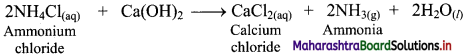ii. Calcium chloride is obtained as an important by-product in this reaction.

Question 54.
Why potassium carbonate cannot be obtained by Solvay process?
Potassium hydrogen carbonate (KHCO3) is highly water soluble and cannot be precipitated out by reacting with potassium choride (KCl) and hence, potassium carbonate (K2CO3) cannot be obtained by Solvay process.Question 55.
What is the action of heat on crystalline sodium carbonate (washing soda)?
i. On heating washing soda (decahydrate of sodium carbonate) up to 373 K, it loses water molecules to form corresponding monohydrate.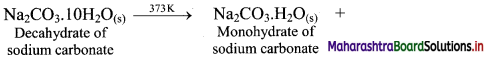ii. On heating above 373 K, monohydrate further loses water and changes into white anhydrous powder called soda ash.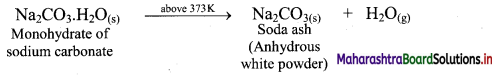Question 56.
Give reason: Aqueous solution of sodium carbonate is alkaline in nature.
i. Sodium carbonate is hydrolysed by water as shown in the reaction given below.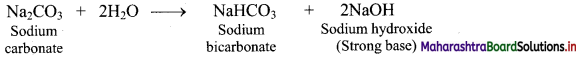ii. One of the products formed as a result of hydrolysis is NaOH which is a strong base.
Hence, aqueous solution of sodium carbonate is alkaline in nature due to formation of strong base (NaOH).

Question 57.
What are the uses of sodium carbonate?
Uses of sodium carbonate:

• Due to its alkaline properties, sodium carbonate has an emulsifying effect on grease and dirt and hence, it is used as a cleaning material.
• It is used to make hard water soft as it precipitates out the soluble calcium and magnesium salts in hard water as carbonates.
For example: Ca(HCO3)2(aq) + Na2CO3(aq) → CaCO3(s) + 2NaHCO3(aq)
• It is used for commercial production of soap and caustic soda.
• Sodium carbonate is used as an important laboratory reagent.

Question 58.
Describe the preparation of sodium hydroxide by Castner-Kellner process.
OR
Explain the electrolysis method for preparation of sodium hydroxide.
i. Sodium hydroxide (caustic soda) is commercially obtained by the electrolysis of aqueous sodium chloride solution (brine) in Castner-Kellner cell (mercury cathode cell).
ii. In Castner-Kellner cell, mercury is used as cathode, carbon rod as anode and brine solution is used as electrolyte which is subjected to electrolysis.

iii. During electrolysis, the following reactions take place:
a. At cathode: Sodium ions get reduced to metallic sodium, which combines with mercury to form sodium amalgam (Na-Hg).
$$\mathrm{Na}^{+}+\mathrm{e}^{-} \stackrel{\mathrm{Hg}}{\longrightarrow} \mathrm{Na} \text {-amalgam }$$
b. At anode: Chloride ions are oxidized and thus, chlorine gas is evolved.
$$\mathrm{Cl}^{-} \longrightarrow \frac{1}{2} \mathrm{Cl}_{2}+\mathrm{e}^{-}$$

iv. Sodium amalgam is then treated with water to obtain sodium hydroxide and hydrogen gas.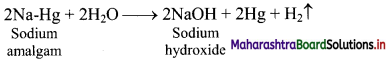Question 59.
Enlist the physical properties of sodium hydroxide.
Physical properties of sodium hydroxide:

• Sodium hydroxide (NaOH) is a white deliquescent solid.
• It has a melting point of 591 K.
• It is highly water soluble and gives a strongly alkaline solution.
• The surface of sodium hydroxide solution absorbs atmospheric CO2 to form Na2CO3.Question 60.
Explain how sodium hydroxide is commercially important.
Commercial uses of sodium hydroxide:

• Sodium hydroxide is used in purification of bauxite (the aluminium ore).
• It is used in commercial production of soap, paper, artificial silk and many chemicals.
• It is used for mercerising cotton fabrics.
• It is used in petroleum refining.
• It is also used as an important laboratory reagent.

Question 61.
Calcium carbonate occurs naturally in which forms?
Calcium carbonate (CaCO3) occur naturally in the form of chalk, limestone and marble.

Question 62.
Describe the various methods used for preparation of calcium carbonate.
i. a. Calcium carbonate is prepared by passing carbon dioxide through solution of calcium hydroxide (slaked lime). This results in the formation of water insoluble solid calcium carbonate.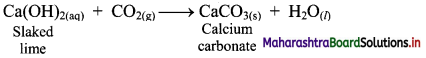b. However, excess carbon dioxide transforms the precipitate of CaCO3 into water-soluble calcium bicarbonate and therefore, it has to be avoided.

ii. Calcium carbonate can also be prepared by adding solution of calcium chloride to a solution of sodium carbonate. This results in the formation of calcium carbonate as precipitate.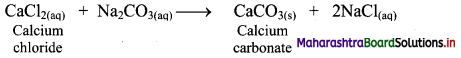Question 63.
Why controlled addition of CO2 is essential during preparation of calcium carbonate from slaked lime?
When excess of CO2 is present, it leads to the formation of water-soluble calcium bicarbonate.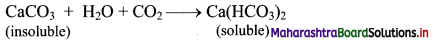Hence, while preparing calcium carbonate from slaked lime, controlled addition of CO2 is essential.

Question 64.
Mention some physical properties of calcium carbonate.

• Calcium carbonate is soft, light, white powder.
• It is practically insoluble in water.Question 65.
What happens when:
i. calcium carbonate is thermally decomposed?
ii. calcium carbonate reacts with dilute mineral acids?
i. When calcium carbonate is heated to 1200 K, it decomposes into calcium oxide along with evolution of carbon dioxide gas.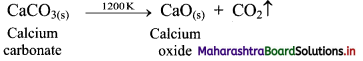ii. Calcium carbonate reacts with dilute mineral acids such as HCl and H2SO4 to give the corresponding calcium salt and liberate carbon dioxide gas.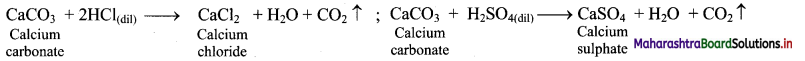Question 66.
Give the important uses of calcium carbonate.

• Calcium carbonate in the form of marble is used as building material.
• It is used in the manufacture of quicklime (CaO) which is the major ingredient of cement.
• A mixture of CaCO3 and MgCO3 is used as flux in the extraction of metals from their ores.
• It is required for the manufacture of high-quality paper.
• It is an important ingredient in toothpaste, chewing gum, dietary supplements of calcium and filler in cosmetics.

Question 67.
Match the pairs.

 Column A Column B i. Castner-Kellner cell a. Na2CO ii. Slaked lime b. CaCO3 iii. Solvay process c. NaOH iv. Limestone d. Ca(OH)2

Ans:
i – c,
ii – d,
iii – a,
iv – b

Question 68.
How is hydrogen peroxide prepared by the action of cold dilute H2SO4 on
i. Hydrated barium peroxide?
ii. sodium peroxide (Merck process)?
Preparation of hydrogen peroxide by the action of cold dilute H2SO4 on
i. hydrated barium peroxide: When hydrated barium peroxide is treated with ice-cold dilute sulphuric acid, the precipitate of barium sulphate is obtained. This precipitate is then filtered off to get hydrogen peroxide solution.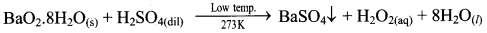ii. Sodium peroxide (Merck process): When small quantity of sodium peroxide is added to ice-cold solution of dilute sulphuric acid with stirring, it gives hydrogen peroxide.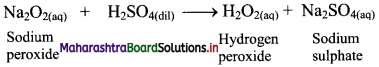Question 69.
Explain how hydrogen peroxide can be obtained by electrolysis method.
i. H2O2 can be manufactured by electrolysis of 50% H2SO4. In this method, 50% solution of H2SO4 is subjected to an electrolytic oxidation to form peroxydisuiphuric acid at anode.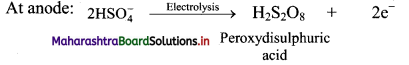ii. On hydrolysis, peroxy sulphuric yields hydrogen peroxide.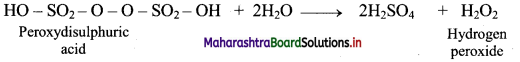iii. This method can be used for the laboratory preparation of D2O2.Question 70.
Describe the industrial method for preparation of hydrogen peroxide.
OR
How is hydrogen peroxide obtained from 2-ethylanthraquinol?
i. Industrially hydrogen peroxide is prepared by air-oxidation of 2-ethylanthraquinol.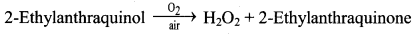ii. 2-Ethylanthraquinone is reduced back to 2-ethylanthraquinol by catalytic hydrogenation.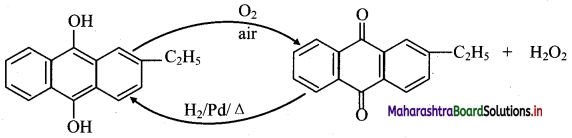Question 71.
What are the physical properties of hydrogen peroxide?

• Pure H2O2 is a very pale blue coloured liquid.
• Its boiling point is 272.4 K.
• H2O2 is miscible in water and forms a hydrate (H2O2. H2O).

Question 72.
How is strength of H2O2 solution expressed?

• Strength of aqueous solution of H2O2 is expressed in ‘volume’ units i.e., volume strength.
• The commercially marketed 30% (by mass) solution of H2O2 has volume strength of 100 volume.
• It means that 1 mL of 30% solution of H2O2 will give 100 mL oxygen at STP.

Thus, Volume strength refers to the volume of oxygen (O2) in litres at STP obtained by decomposition of 1 litre of the sample.

Question 73.
Write reactions depicting oxidising and reducing action of hydrogen peroxide in acidic medium.
H2O2 acts as a mild oxidising as well as reducing agent.
i. Oxidising action of H2O2 in acidic medium.
$$2 \mathrm{Fe}_{(\mathrm{aq})}^{2+}+2 \mathrm{H}_{(\mathrm{aq})}^{+}+\mathrm{H}_{2} \mathrm{O}_{2(\mathrm{aq})} \longrightarrow 2 \mathrm{Fe}_{(\mathrm{aq})}^{3+}+2 \mathrm{H}_{2} \mathrm{O}_{(l)}$$

ii. Reducing action of H2O2 in acidic medium.
$$2 \mathrm{MnO}_{4}^{-}+6 \mathrm{H}^{+}+5 \mathrm{H}_{2} \mathrm{O}_{2} \rightarrow 2 \mathrm{Mn}^{2+}+8 \mathrm{H}_{2} \mathrm{O}+5 \mathrm{O}_{2}$$

Question 74.
Enlist uses of hydrogen peroxide.

• Hydrogen peroxide is used as mouthwash, germicide and mild antiseptic.
• It is used as a preservative for milk and wine.
• It is used as a bleaching agent for soft materials, due to its mild oxidising property.
• Due to its reducing property, is used as an antichlor to remove excess chlorine from fabrics which have been bleached by chlorine.
• Nowadays it is used in environmental chemistry for pollution control and restoration of aerobic condition of sewage water.Question 75.
Describe preparation and properties of lithium aluminium hydride (LAH).
i. Preparation: Lithium hydride when treated with aluminium chloride, gives lithium aluminium hydride, (LiAlH4).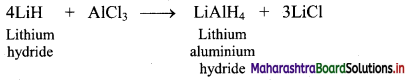ii. Properties:

• Lithium aluminium hydride is a colourless solid.
• It reacts violently with water and even with atmospheric moisture.

Question 76.
How is lithium aluminium hydride (LAH) useful in organic synthesis?
i. LAH is a source of hydride (H) and therefore, it is used as a reducing agent in organic synthesis.
For example: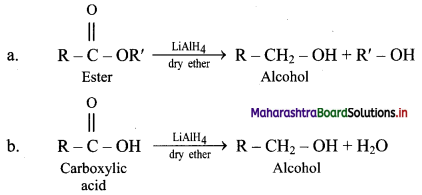ii. It is useful in the preparation of PH3 (phosphine).
4PCl3 + 3LiAlH4 → 4PH2 + AlCl3 + LiCl

Question 77.
Complete the following reactions by mentioning the reagent/reaction conditions under which these reactions are carried out.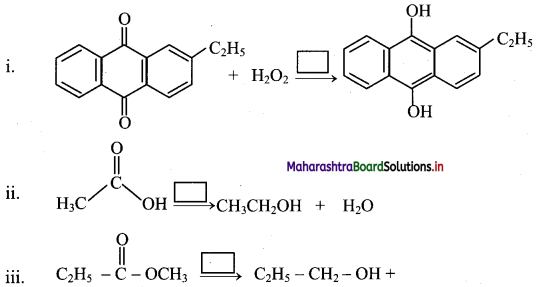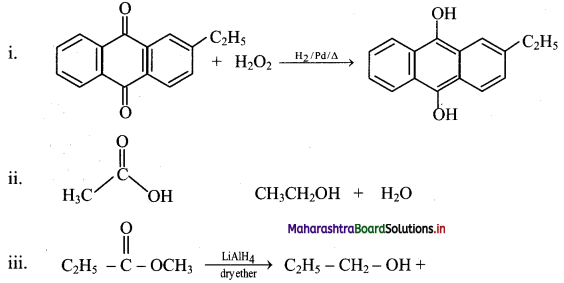Question 78.
Calculate % (by mass) of a H2O2 solution which is 45.4 volume.
Given: 45.4 volume H2O2 solution
To find: % (by mass) of H2O2
Formula: Percentage (%) by mass = $$\frac{\text { Mass of solute }}{\text { Mass of solution }} \times 100$$
calculation: 45.4 volume H2O2 solution means 1 L of this solution will liberate 45.4 L of O2 at STP.
Hydrogen peroxide (H2O2) decomposes as: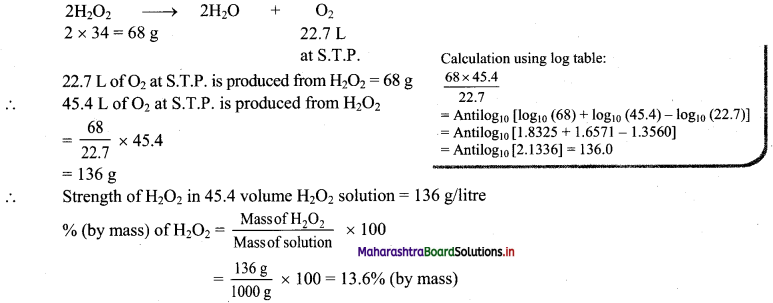Ans: % (by mass) of H2O2 in 45.4 volume H2O2 solution is 13.6%.

Question 79.
Calculate the strength (g/L) of 20 volume solution of hydrogen peroxide.
Solution:
Given: 20 volume H2O2 solution
To find: Strength of H2O2 (g/L)
Formula: 20 volume H2O2 solution means that 1 L of this solution will liberate 20 L of oxygen at S.T.P. Let us calculate the amount of H2O2 (in grams) which gives 20 L of oxygen at S.T.P. This amount will be present in 1 L of 20 volume solution of H2O2.
Hydrogen peroxide (H2O2) decomposes as: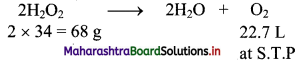22.7 L of O2 at S.T.P. is produced from H2O2 = 68 g
20 L of O2 at S.T.P. is produced from H2O2 = $$\frac {68}{22.7}$$ × 20 = 59.912g = 59.912 g/litre
Ans: Strength of H2O2 in 20 volume H2O2 solution is 59.912 g/L.Question 80.
Calculate the volume strength of a 5% solution of hydrogen peroxide.
Solution:
Given: 5% solution of H2O2
To find: Volume strength of H2O2 solution
Calculation: 100 mL of solution contains 5 g of H2O2
1000 mL of solution will contain $$\frac {5}{100}$$ × 1000 = 50 g of H2O2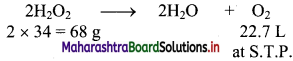68 g of H2O2 will give O2 at S.T.P. = 22.7 L
50 g of H2O2 is present in 1000 mL of H2O2 or 1 L of H2O2
50 g of H2O2 will give O2 at S.T.P. = $$\frac {22.7}{68}$$ × 50 = 16.691 L
∴ 1 L of H2O2 gives O2 at S.T.P. = 16.691 L
∴ Strength of H2O2 = 16.691 volume
Ans: The given 5% H2O2 solution is equivalent to 16.691 volume solution of hydrogen peroxide.

Question 81.
Naina is a school going kid. Every morning her mother makes her drink a glass of milk. When she asked her mother that why she has to drink a glass of milk daily, her mother told her that it is beneficial in maintaining healthy bones and teeth. Regular consumption of milk is recommended because it is a rich source of calcium.
i. In which form is calcium important for bones and teeth?
ii. Calcium belongs to which family in the modern periodic table?
iii. Write its electronic configuration.
iv. Calcium contain how many valence electrons?
v. Give any two-biological importance of calcium.
i. Calcium is important for bones and teeth in the form of apatite [Ca(PO4)2].
ii. It belongs to the family of alkaline earth metals in the modem periodic table.
iii. Electronic configuration of 20Ca is 1s2 2s2 2p6 3s2 3p6 4s2.
iv. Calcium contains two valence electrons as it has two electrons in its outermost shell (4s).

v. Calcium ion (Ca2+)

• Ca2+ ions are important for bones and teeth in the fonn of apatite [Ca3(PO4)2].
• They play an important role in blood clotting.
• Ca2+ ions are required for contraction and stretching of muscles.
• They are also required to maintain the regular beating of heart.

Multiple Choice Questions

1. Of all the elements present in the periodic table, ………… has the simplest atomic structure.
(A) lithium
(B) beryllium
(C) hydrogen
(D) helium
(C) hydrogen

2. Electronic configuration of hydrogen is similar to that of ……………
(A) transition elements
(B) inert gases
(C) alkaline earth metals
(D) alkali metals
(D) alkali metals3. Isotopes are atoms of the same element having different ………….. number.
(A) neutron
(B) proton
(C) electron
(D) Both (A) and (B)
(A) neutron

4. Tritium, $$\left({ }_{1}^{3} \mathrm{H}\right)$$ ……………
(A) is an isotope of hydrogen
(B) contains one electron, one proton and two neutrons
(C) is a beta particle emitter
(D) all of these
(D) all of these

5. In the electrolysis of acidified water using, ………….. is liberated at the anode.
(A) dihydrogen
(B) sulphate ions
(C) oxygen
(D) chloride ions
(C) oxygen

6. Water gas is a mixture of ………….
(A) CO + H2
(B) CO2 + H2
(C) O2 + H2
(D) CO + O2
(A) CO + H2

7. During production of dihydrogen by water-gas shift reaction, which of the following is present as an impurity?
(A) Carbon monoxide
(B) Carbon dioxide
(C) Calcium carbonate
(D) Calcium oxide
(B) Carbon dioxide8. Solution is used to remove carbon dioxide present in the mixture along with dihydrogen.
(A) Sodium hydroxide
(B) Hydrochloric acid
(C) Magnesium chloride
(D) Sodium arsenite
(D) Sodium arsenite

9. The reaction between dihydrogen and …………… is highly exothermic.
(A) halogens
(B) dioxygen
(C) dinitrogen
(D) metals
(B) dioxygen

10. The elements of group 1 and group 2 belong to which block of the modem periodic table?
(A) d-block
(B) s-block
(C) p-block
(D) f-block
(B) s-block

11. Which of the following is NOT an alkaline earth metal?
(A) Beryllium
(B) Barium
(C) Calcium
(D) Caesium
(D) Caesium

12. The common oxidation state for alkali metals is …………….
(A) +2
(B) +1
(C) +3
(D) +4
(B) +1

13. All alkaline earth metals have ………….. valence electrons in the outermost orbit.
(A) one
(B) two
(C) three
(D) four
(B) two14. Electronic configuration of potassium with respect to nearest noble gases is …………..
(A) [He]2s1
(B) [Ne]3s1
(C) [Ar]4s1
(D) [Kr]5s1
(C) [Ar]4s1

15. Which of the following is radioactive alkali metal?
(A) Rubidium
(B) Caesium
(C) Francium
(D) Beryllium
(C) Francium

16. Which of the following element is rarest amongst s-block elements?
(A) Strontium
(B) Barium
(D) Calcium

17. Which of the following is FALSE?
(A) Alkali metals readily loose electron to form monovalent M+ ions.
(B) In a group, from Li to Cs, atomic and ionic radii increase with atomic number.
(C) The monovalent ions of alkali metals are larger in size than the parent atoms.
(D) Ionization enthalpies decrease down the group from Li to Cs.
(C) The monovalent ions of alkali metals are larger in size than the parent atoms.

18. The first ionization enthalpies of alkaline earth metals are …………. than those of the corresponding alkali metals.
(A) higher
(B) lower
(C) same
(D) none of these
(A) higher

19. Which of the following alkaline earth metal does NOT react with water?
(A) Sr
(B) Mg
(C) Ca
(D) Be
(D) Be20. Oxides and hydroxides of alkaline earth metals except beryllium are ………….. in nature.
(A) acidic
(B) basic
(C) amphoteric
(D) neutral
(B) basic

21. ………… is an excellent absorbent of carbon dioxide.
(A) KO2
(B) KCl
(C) KOH
(D) KHCO3
(A) KO2

22. Lithium shows diagonal relationship with ……………
(A) beryllium
(B) magnesium
(C) calcium
(D) boron
(B) magnesium

23. The diagonal relationship between Li and Mg is due to the similarity in ……………
(A) ionic sizes
(B) electronegativity value
(C) polarizing power
(D) all of the above
(D) all of the above

24. The alkali metal that reacts with nitrogen directly to form nitride is ……………
(A) Li
(B) Na
(C) K
(D) Rb
(A) Li

25. In the Solvay process, the chief products are ……………
(A) CaCO3 and Ca(HCO3)2
(B) Na2CO3 and NaHCO3
(C) Na2SO4 and NaHSO4
(D) CaCl2 and Ca(NO3)2
(B) Na2CO3 and NaHCO326. In Castner-Kellner process for preparation of sodium hydroxide, ………….. is subjected to electrolysis.
(A) NaCl
(B) NaOH
(C) Na2O
(D) Na2CO3